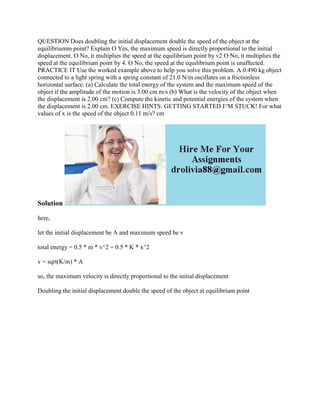Ce diaporama a bien été signalé.
Le téléchargement de votre SlideShare est en cours. ×

# QUESTION Does doubling the initial displacement double the speed of th.docx

Publicité
Publicité
Publicité
Publicité
Publicité
Publicité
Publicité
Publicité
Publicité
Publicité
PublicitéChargement dans…3
×

## Consultez-les par la suite

1 sur 2 Publicité

# QUESTION Does doubling the initial displacement double the speed of th.docx

QUESTION Does doubling the initial displacement double the speed of the object at the equilibriumm point? Explain O Yes, the maximum speed is directly proportional to the initial displacement. O No, it multiplies the speed at the equilibrium point by v2 O No, it multiplies the speed at the equilibrium point by 4. O No, the speed at the equilibrium point is unaffected. PRACTICE IT Use the worked example above to help you solve this problem. A 0.490 kg object connected to a light spring with a spring constant of 21.0 N/m oscillates on a frictionless horizontal surface. (a) Calculate the total energy of the system and the maximum speed of the object if the amplitude of the motion is 3.00 cm m/s (b) What is the velocity of the object when the displacement is 2.00 cm? (c) Compute the kinetic and potential energies of the system when the displacement is 2.00 cm. EXERCISE HINTS: GETTING STARTED I\'M STUCK! For what values of x is the speed of the object 0.11 m/s? cm
Solution
here,
let the initial displacement be A and maximum speed be v
total energy = 0.5 * m * v^2 = 0.5 * K * x^2
v = sqrt(K/m) * A
so, the maximum velocity is directly proportional to the initial displacement
Doubling the initial displacement double the speed of the object at equilibrium point
.

QUESTION Does doubling the initial displacement double the speed of the object at the equilibriumm point? Explain O Yes, the maximum speed is directly proportional to the initial displacement. O No, it multiplies the speed at the equilibrium point by v2 O No, it multiplies the speed at the equilibrium point by 4. O No, the speed at the equilibrium point is unaffected. PRACTICE IT Use the worked example above to help you solve this problem. A 0.490 kg object connected to a light spring with a spring constant of 21.0 N/m oscillates on a frictionless horizontal surface. (a) Calculate the total energy of the system and the maximum speed of the object if the amplitude of the motion is 3.00 cm m/s (b) What is the velocity of the object when the displacement is 2.00 cm? (c) Compute the kinetic and potential energies of the system when the displacement is 2.00 cm. EXERCISE HINTS: GETTING STARTED I\'M STUCK! For what values of x is the speed of the object 0.11 m/s? cm
Solution
here,
let the initial displacement be A and maximum speed be v
total energy = 0.5 * m * v^2 = 0.5 * K * x^2
v = sqrt(K/m) * A
so, the maximum velocity is directly proportional to the initial displacement
Doubling the initial displacement double the speed of the object at equilibrium point
.

Publicité
Publicité

### QUESTION Does doubling the initial displacement double the speed of th.docx

1. 1. QUESTION Does doubling the initial displacement double the speed of the object at the equilibriumm point? Explain O Yes, the maximum speed is directly proportional to the initial displacement. O No, it multiplies the speed at the equilibrium point by v2 O No, it multiplies the speed at the equilibrium point by 4. O No, the speed at the equilibrium point is unaffected. PRACTICE IT Use the worked example above to help you solve this problem. A 0.490 kg object connected to a light spring with a spring constant of 21.0 N/m oscillates on a frictionless horizontal surface. (a) Calculate the total energy of the system and the maximum speed of the object if the amplitude of the motion is 3.00 cm m/s (b) What is the velocity of the object when the displacement is 2.00 cm? (c) Compute the kinetic and potential energies of the system when the displacement is 2.00 cm. EXERCISE HINTS: GETTING STARTED I'M STUCK! For what values of x is the speed of the object 0.11 m/s? cm Solution here, let the initial displacement be A and maximum speed be v total energy = 0.5 * m * v^2 = 0.5 * K * x^2 v = sqrt(K/m) * A so, the maximum velocity is directly proportional to the initial displacement Doubling the initial displacement double the speed of the object at equilibrium point